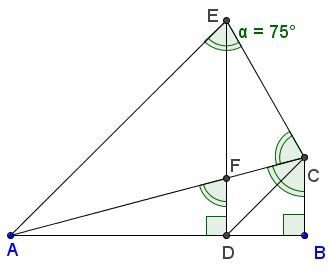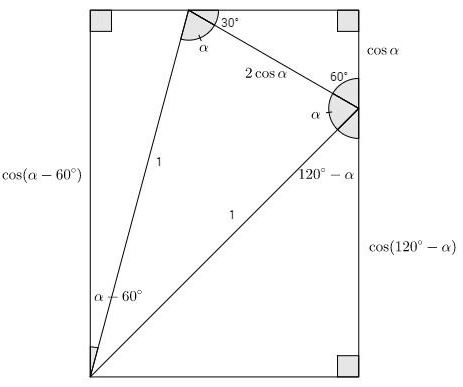# cos 75 + cos 45 = cos 15

### Problem

$\cos 45^{\circ}+\cos 75^{\circ}=\cos 15^{\circ}$

which was posted by Imad Zak to the CutTheKnotMath facebook page along with a solution (Solution 1).

### Solution 1

Using the well known formula $\displaystyle\cos\alpha -\cos\beta=-2\sin\frac{\alpha +\beta}{2}\sin\frac{\alpha-\beta}{2},\;$ we obtain

\displaystyle\begin{align} \cos 15^{\circ}-\cos 75^{\circ} &= -2\sin\frac{15^{\circ}+75^{\circ}}{2}\sin\frac{15^{\circ}-75^{\circ}}{2}\\ &=2\sin 45^{\circ}\sin 30^{\circ}\\ &=2\cdot \frac{\sqrt{2}}{2}\cdot \frac{1}{2}\\ &=\frac{\sqrt{2}}{2}\\ &=\cos 45^{\circ}. \end{align}

### Solution 2

Elsewhere, based on Larry Hoehn's diagram,we obtained

$\displaystyle \cos 75^{\circ}=\sin 15^\circ = \frac{\sqrt{6} - \sqrt{2}}{4}$

and

$\displaystyle \cos 15^\circ = \frac{\sqrt{6} + \sqrt{2}}{4}.$

Thus, $\displaystyle \left( \frac{\sqrt{6} + \sqrt{2}}{4}\right)-\left( \frac{\sqrt{6} - \sqrt{2}}{4}\right)=\frac{\sqrt{2}}{2}=\cos 45^{\circ}.$

Note that the Larry Hoehn's diagram presents a proof without words of this result: indeed, $AC=BC=2\cos 15^{\circ},\;$ $CD=2\cos 75^{\circ},\;$ and, finally, $AD=\sqrt{2}=2\cos 45^{\circ}.\;$ Thus the identity in the title follows from $AD+CD=AC.$

### Solution 3

Here's another proof without words (that may still need a few words of explanation, assisted by angle chasing.) In the diagram, $AC=1,\;$ $BD=BC=\cos 75^{\circ},\;$ $AD=\cos 45^{\circ},\;$ and $AB=\cos 15^{\circ}.$The construction proceeds in the following steps:

1. $\Delta ABC,\;$ with $\angle ABC=90^{\circ}\;$ and $\angle ACB=75^{\circ}.\;$ This makes $\angle BAC=15^{\circ}.$

2. $\Delta ACE,\;$ with $\angle ACE=\angle AEC=75^{\circ}.\;$ This makes $\angle CAE=30^{\circ}\;$ and $\angle DAE=45^{\circ}.$

3. $BD=BC.$

4. $FD\perp AB$ ($F\in AC)\;$ which makes $\angle AFD=75^{\circ}.$

5. $EF',$ $F'\in AC,\;$ with $\angle CEF'=30^{\circ},\;$ which makes $\angle CF'E=75^{\circ},\;$ implying $F=F'.$

The required identity follows from $AD+BD=AB,$

### Solution 4

This PWW is by Dan Lawson:### Generalization

Dan Lawson also supplied a PWW generalization: $\cos\alpha +\cos(120^{\circ}-\alpha )=\cos(\alpha-60^{\circ}):$### Solution 5

This solution was anonymously left in comments:In the diagram, $y=\cos 75^{\circ},\;$ while $x\;$ could be found from the Law of Sines: $\displaystyle\frac{1}{\sin 135^{\circ}}=\frac{x}{\sin 30^{\circ}},\;$ such that $\displaystyle x=\frac{\sqrt{2}}{2}=\cos 45^{\circ}.$

### Solution 6

This is an attempt to present the previous proof without trigonometry:### Trigonometry